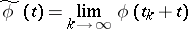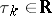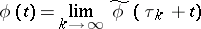# Recurrent function

(diff) ← Older revision | Latest revision (diff) | Newer revision → (diff)

A function that is a recurrent point of the shift dynamical system. An equivalent definition is: A function, whereis a metric space, is called recurrent if it has a pre-compact set of values, is uniformly continuous and if for each sequence of numberssuch that the limitexists (the limit in the compact-open topology, i.e. uniformly on each segment) a sequence of numberscan be found such thatin the compact-open topology.

Ifis a bounded uniformly-continuous function, then numberscan be found such that the limit (in the compact-open topology)exists and is a recurrent function. Every almost-periodic function, and, in particular, every periodic function, is recurrent.

How to Cite This Entry:
Recurrent function. Encyclopedia of Mathematics. URL: http://encyclopediaofmath.org/index.php?title=Recurrent_function&oldid=15003
This article was adapted from an original article by V.M. Millionshchikov (originator), which appeared in Encyclopedia of Mathematics - ISBN 1402006098. See original article